Part of the "Understanding F# types" series (link)

# Tuples

Multiplying types together

We’re ready for our first extended type – the tuple.

Let’s start by stepping back again and looking at a type such as “int”. As we hinted at before, rather than thinking of “int” as a abstract thing, you can think of it as concrete collection of all its possible values, namely the set {…,-3, -2, -1, 0, 2, 3, …}.

So next, imagine two copies of this “int” collection. We can “multiply” them together by taking the Cartesian product of them; that is, making a new list of objects by picking every possible combination of the two “int” lists, as shown below: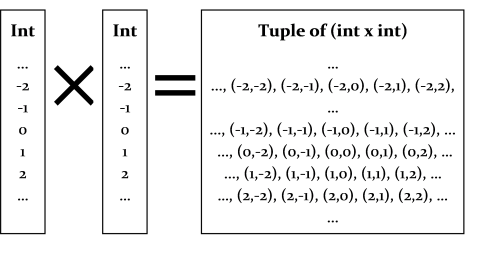As we have already seen, these pairs are called tuples in F#. And now you can see why they have the type signature that they do. In this example, the “int times int” type is called “`int * int`”, and the star symbol means “multiply” of course! The valid instances of this new type are all the pairs: (-2,2),(-1,0), (2,2) and so on.

Let’s see how they might be used in practice:

``````let t1 = (2,3)
let t2 = (-2,7)
``````

Now if you evaluate the code above you will see that the types of t1 and t2 are `int*int` as expected.

``````val t1 : int * int = (2, 3)
val t2 : int * int = (-2, 7)
``````

This “product” approach can be used to make tuples out of any mixture of types. Here is one for “int times bool”.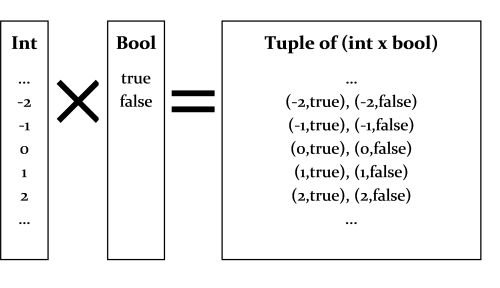And here is the usage in F#. The tuple type above has the signature “`int*bool`”.

``````let t3 = (2,true)
let t4 = (7,false)

// the signatures are:
val t3 : int * bool = (2, true)
val t4 : int * bool = (7, false)
``````

Strings can be used as well, of course. The universe of all possible strings is very large, but conceptually it is the same thing. The tuple type below has the signature “`string*int`”.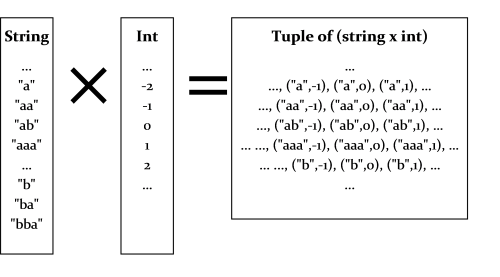Test the usage and signatures:

``````let t5 = ("hello",42)
let t6 = ("goodbye",99)

// the signatures are:
val t5 : string * int = ("hello", 42)
val t6 : string * int = ("goodbye", 99)
``````

And there is no reason to stop at multiplying just two types together. Why not three? Or four? For example, here is the type `int * bool * string`.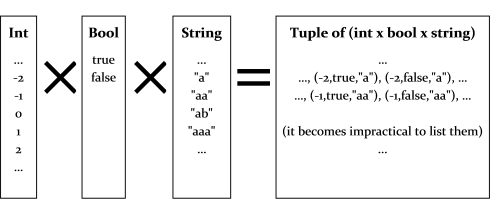Test the usage and signatures:

``````let t7 = (42,true,"hello")

// the signature is:
val t7 : int * bool * string = (42, true, "hello")
``````

## Generic tuples

Generics can be used in tuples too.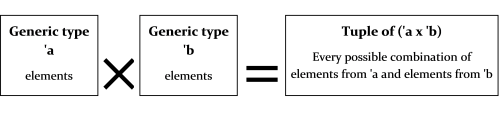The usage is normally associated with functions:

``````let genericTupleFn aTuple =
let (x,y) = aTuple
printfn "x is %A and y is %A" x y
``````

And the function signature is:

``````val genericTupleFn : 'a * 'b -> unit
``````

which means that “`genericTupleFn`” takes a generic tuple `('a * 'b)` and returns a `unit`

## Tuples of complex types

Any kind of type can be used in a tuple: other tuples, classes, function types, etc. Here are some examples:

``````// define some types
type Person = {First:string; Last:string}
type Complex = float * float
type ComplexComparisonFunction = Complex -> Complex -> int

// define some tuples using them
type PersonAndBirthday = Person * System.DateTime
type ComplexPair = Complex * Complex
type ComplexListAndSortFunction = Complex list * ComplexComparisonFunction
type PairOfIntFunctions = (int->int) * (int->int)
``````

Some key things to know about tuples are:

• A particular instance of a tuple type is a single object, similar to a two-element array in C#, say. When using them with functions they count as a single parameter.
• Tuple types cannot be given explicit names. The “name” of the tuple type is determined by the combination of types that are multiplied together.
• The order of the multiplication is important. So `int*string` is not the same tuple type as `string*int`.
• The comma is the critical symbol that defines tuples, not the parentheses. You can define tuples without the parentheses, although it can sometimes be confusing. In F#, if you see a comma, it is probably part of a tuple.

These points are very important – if you don’t understand them you will get confused quite quickly!

And it is worth re-iterating the point made in previous posts: don’t mistake tuples for multiple parameters in a function.

``````// a function that takes a single tuple parameter
// but looks like it takes two ints
let addConfusingTuple (x,y) = x + y
``````

## Making and matching tuples

The tuple types in F# are somewhat more primitive than the other extended types. As you have seen, you don’t need to explicitly define them, and they have no name.

It is easy to make a tuple – just use a comma!

``````let x = (1,2)
let y = 1,2        // it's the comma you need, not the parentheses!
let z = 1,true,"hello",3.14   // create arbitrary tuples as needed
``````

And as we have seen, to “deconstruct” a tuple, use the same syntax:

``````let z = 1,true,"hello",3.14   // "construct"
let z1,z2,z3,z4 = z           // "deconstruct"
``````

When pattern matching like this, you must have the same number of elements, otherwise you will get an error:

``````let z1,z2 = z     // error FS0001: Type mismatch.
// The tuples have differing lengths
``````

If you don’t need some of the values, you can use the “don’t care” symbol (the underscore) as a placeholder.

``````let _,z5,_,z6 = z     // ignore 1st and 3rd elements
``````

As you might guess, a two element tuple is commonly called a “pair” and a three element tuple is called a “triple” and so on. In the special case of pairs, there are functions `fst` and `snd` which extract the first and second element.

``````let x = 1,2
fst x
snd x
``````

They only work on pairs. Trying to use `fst` on a triple will give an error.

``````let x = 1,2,3
fst x              // error FS0001: Type mismatch.
// The tuples have differing lengths of 2 and 3
``````

## Using tuples in practice

Tuples have a number of advantages over other more complex types. They can be used on the fly because they are always available without being defined, and thus are perfect for small, temporary, lightweight structures.

### Using tuples for returning multiple values

It is a common scenario that you want to return two values from a function rather than just one. For example, in the `TryParse` style functions, you want to return (a) whether the value was parsed and (b) if parsed, what the parsed value was.

Here is an implementation of `TryParse` for integers (assuming it did not already exist, of course):

``````let tryParse intStr =
try
let i = System.Int32.Parse intStr
(true,i)
with _ -> (false,0)  // any exception

//test it
tryParse "99"
tryParse "abc"
``````

Here’s another simple example that returns a pair of numbers:

``````// return word count and letter count in a tuple
let wordAndLetterCount (s:string) =
let words = s.Split [|' '|]
let letterCount = words |> Array.sumBy (fun word -> word.Length )
(words.Length, letterCount)

//test
wordAndLetterCount "to be or not to be"
``````

### Creating tuples from other tuples

As with most F# values, tuples are immutable and the elements within them cannot be assigned to. So how do you change a tuple? The short answer is that you can’t – you must always create a new one.

Say that you need to write a function that, given a tuple, adds one to each element. Here’s an obvious implementation:

``````let addOneToTuple aTuple =
let (x,y,z) = aTuple
(x+1,y+1,z+1)   // create a new one

// try it
``````

This seems a bit long winded – is there a more compact way? Yes, because you can deconstruct a tuple directly in the parameters of a function, so that the function becomes a one liner:

``````let addOneToTuple (x,y,z) = (x+1,y+1,z+1)

// try it
``````

### Equality

Tuples have an automatically defined equality operation: two tuples are equal if they have the same length and the values in each slot are equal.

``````(1,2) = (1,2)                      // true
(1,2,3,"hello") = (1,2,3,"bye")    // false
(1,(2,3),4) = (1,(2,3),4)          // true
``````

Trying to compare tuples of different lengths is a type error:

``````(1,2) = (1,2,3)                    // error FS0001: Type mismatch
``````

And the types in each slot must be the same as well:

``````(1,2,3) = (1,2,"hello")   // element 3 was expected to have type
// int but here has type string
(1,(2,3),4) = (1,2,(3,4)) // elements 2 & 3 have different types
``````

Tuples also have an automatically defined hash value based on the values in the tuple, so that tuples can be used as dictionary keys without problems.

``````(1,2,3).GetHashCode()
``````

### Tuple representation

And as noted in a previous post, tuples have a nice default string representation, and can be serialized easily.

``````(1,2,3).ToString()
``````